>  Chemistry Test - 2

# Chemistry Test - 2

Test Description

## 45 Questions MCQ Test | Chemistry Test - 2

 1 Crore+ students have signed up on EduRev. Have you?
Chemistry Test - 2 - Question 1

### Calorific value of hydrogen gas is –143 kJ g-1. The standard enthalpy of formation of H2O will be

Chemistry Test - 2 - Question 2

###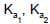and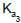are the three dissociation constants of a triprotic acid. Their correct order is represented as

Chemistry Test - 2 - Question 3

### For the A → B process Given : ΔH = +ve,ΔS = -ve, TΔS > ΔH. which of the following statement is correct ?

Chemistry Test - 2 - Question 4

Which of the following statements regarding water is not correct ?

Chemistry Test - 2 - Question 5

Which one of the following equations represents a redox reaction ?

Chemistry Test - 2 - Question 6

The standard heat of formation of NO2(g) and N2O4(g) are 8.0 and 2.0 kcal mol-1 respectively. The heat of dimerizatioon of NO2 in kcal is

Chemistry Test - 2 - Question 7

In the reaction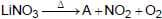; A is

Chemistry Test - 2 - Question 8

Ionic radii of alkali metal ions in water are in the order

Chemistry Test - 2 - Question 9

Correctly matched pairs are
Metal                    Colour of the flame
1. Lithium              Red
2. Barium              Green
3. Potassium         Violet
4. Rubidium           Yellow

Chemistry Test - 2 - Question 10

For making H2O2 in the laboratory

Chemistry Test - 2 - Question 11

500 mL vessel contains 1.5 M each of A, B, C and D at equilibrium. If 0.5 M each of C and D are taken out, the value of Kc for A + B → C + D , will be

Chemistry Test - 2 - Question 12

The solubility of silver carbonate can be found out by the formula

Chemistry Test - 2 - Question 13

Which of the following solutions is competent enough to cause the largest change in pH, when added to 50 cc of 1 M NaOH solution ?

Chemistry Test - 2 - Question 14

What is false statement regarding ‘10 volume’ H2O2?

Chemistry Test - 2 - Question 15

In which of the following reactions H2O2 acts as a reductant ?

Chemistry Test - 2 - Question 16

Formula to evaluate the pH of KCN solution of concentration C is

Chemistry Test - 2 - Question 17

Which of the following statements is not correct ?

Chemistry Test - 2 - Question 18

An example of a covalent hydride is

Chemistry Test - 2 - Question 19

N2 (g) + O2 (g) → 2NO(g); ΔH = +21.5kcal . For the chemical equilibrium what are the favourable conditions for the more yield of NO ?

Chemistry Test - 2 - Question 20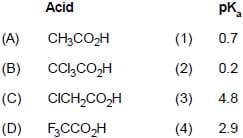Chemistry Test - 2 - Question 21

Which of the following physical property is wrongly expressed ?

Chemistry Test - 2 - Question 22

From the following data, calculate the heat of neutralization of NH3(aq)and HCl(aq)
(i) NH3 (g) + aq.→ NH3 (aq) + 8.4kcal
(ii) HCl(g) + aq. → HCl(aq) + 17.8kcal
(iii) NH3(g) + HCl(g) + aq → NH4Cl + (aq) + 38.2 kcal

Chemistry Test - 2 - Question 23

Which one of the following oxides is most stable ? The equilibrium constants are given at the same temperature

Chemistry Test - 2 - Question 24

Choose the set of the coefficient that correctly balance the equation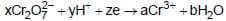Chemistry Test - 2 - Question 25

+1 oxidation number of phosphorus will be shown by the species

Chemistry Test - 2 - Question 26

For the reaction N2(g) + 3H2(g) → 2NH3 initially N2 and H2 were taken in the mole ratio of 1 : 3. At the point of equilibrium 50% of each reactant has been reacted. If the total pressure at equilibrium was P. The partial pressure of NH3 will be

Chemistry Test - 2 - Question 27

Adding inert gas to system, N2 + 3H2 → 2NH3 at equilibrium at constant volume will be

Chemistry Test - 2 - Question 28

NH4COONH2(s) → 2NH3(g) + CO2(g) .If equilibrium pressure is 3 atm for the above reaction, kp for the reaction is

Chemistry Test - 2 - Question 29

Kw = 10-14 (at 250 ) for a distilled water. In order to increase the value of Kw we should

Chemistry Test - 2 - Question 30

Kb for NH4OH is 1.8 x 10-5 , what is the [OH]- in its solution of 0.1 M concentration ?

Chemistry Test - 2 - Question 31

Which of the following statements is not correct ?

Chemistry Test - 2 - Question 32

The oxidation number of an oxidant in a redox reaction

Chemistry Test - 2 - Question 33

Calculate the value of dissociation constant of NH4OH. If the pH of buffer solution containing 0.5 M NH4OH and 0.5 M NH4Cl is 9.0

Chemistry Test - 2 - Question 34

The reaction between thiocyanate and ferric ion is represented by the reactioon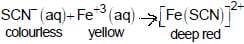Addition of thiocyanate ion to the equilibrium mixture will make

Chemistry Test - 2 - Question 35

ΔH for the reaction ;
Ag2O(s) → 2Ag(s) + 1/2O2(g) is 30.0 kJ mol-1 and ΔS = 0.06 kJK-1 mol-1(at 1 atm pressure). Calculate that temperature at which ΔG = 0 ?

Chemistry Test - 2 - Question 36

What is true for an endothermic spontaneous reaction ?

Chemistry Test - 2 - Question 37

Heat of combustion of carbon is 394 kJ. The heat involved in the combustion of 6.02 x1021 carbon atoms will be

Chemistry Test - 2 - Question 38

Which of the following statements is not correct ?

Chemistry Test - 2 - Question 39

Oxidation state of oxygen in KO3, Na2O2 is

Chemistry Test - 2 - Question 40

A gas absorbs 200 J of heat and expands by 500 cm3 against a constant pressure of 2 x105 Nm-2 .The change in internal energy of the gas will be

Chemistry Test - 2 - Question 41

Given
C(s) + O2(g) → CO2(g);ΔH = -395 kJ
S(s) + O2(g) → SO2(g);ΔH = -295 kJ
CS2(l) + 3O2(g) → CO2(g) + 2SO2(g);ΔH = -1110 kJ

From the above data the heat of formation of CS2 (l) will be

Chemistry Test - 2 - Question 42

Which of the following is known as Indian salt petre?

Chemistry Test - 2 - Question 43

One mole of KMnO4 oxidises sufficient quantity of HCl. How many moles of chlorine gas will be liberated in the process ?

Chemistry Test - 2 - Question 44

Which one of the following equations represents a redox reaction ?

Chemistry Test - 2 - Question 45

The metal used in the photoelectric cell is

 Use Code STAYHOME200 and get INR 200 additional OFF Use Coupon Code